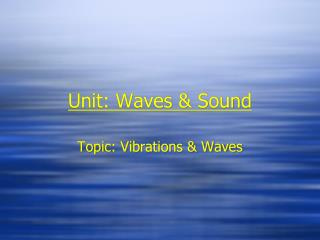DownloadDownload PresentationUnit: Waves & Sound

Unit: Waves & Sound

Download PresentationUnit: Waves & Sound

- - - - - - - - - - - - - - - - - - - - - - - - - - - E N D - - - - - - - - - - - - - - - - - - - - - - - - - - -
Presentation Transcript

1. Unit: Waves & Sound Topic: Vibrations & Waves

2. Question • How many of you know about periodic motion? • It is everywhere in the world - your heart - your breathing - drinking orange juice - What else?

3. Topic: Vibrations & Waves

4. Explain… • Observe… • Explain…

5. Where have you seen waves before?

6. Waves Wave Types: • Mechanical (through media) • Ocean waves • Sound Waves • Flapping Flag • Electromagnetic (through space) • Light • X-ray • Ultraviolet • Infrared • Radio waves • Microwaves

7. Waves Waves transfer energy over a distance in the form of a disturbance. This disturbance can be caused by the vibration of an object. A vibrating object has a ‘rest’ position and will periodically shift from this state to another. Think of the type of vibrational motion exhibited in the following examples.

8. A weight hanging from a spring. A weight hanging from a rope and the rope is twisted. A pendulum. A piece of wood floating in the ocean. Periodic motion occurs when an object repeats a pattern of motion. The vibration, or oscillation is repeated over and over with the same time interval.

9. Types of Vibrations There are 3 basic types of vibrations: transverse vibrations, longitudinal vibrations, and torsionalvibrations. A transverse vibration occurs when an object vibrates perpendicular to its axis at the normal rest position (such a pendulum). A longitudinal vibration occurs when an object travels parallel to its axis at the rest position.

10. A torsional vibration occurs when an object twists around its axis at the rest position, for example a human twisting to the left or the right periodically. Observe the twist to the left, then the rest position, then the twist to the right.

11. Measuring Vibrations • All waves are caused by vibrations • f = # of cycles / ∆t • Frequency is measured in Hertz (Hz) • 1 Hz = 1 cycle / second

12. Cycle • 1 cycle = the time it takes to go from here • To here • And then back again

13. Period • Period is the amount of time for a single vibration to occur. • T= (1/f), T= (Δt)/(# of cycles) • Period is measured in seconds

14. The distance in either direction from the equilibrium, or rest position to maximum displacement is called the amplitude (A) of vibration. A A

15. Objects that are ‘in phase’ vibrate with the same period and pass through the rest position at the same time and in the same direction. In Phase In Phase Out of phase

16. In small groups • I would like you to illustrate to me one of the types of vibrations you learned today with any of the materials/resources you have in this class…. • Calculate the following: - the frequency - the period You will make up the # of cycles & time you so desire 1 person can be a timer 1 person can be the person who counts the cycles 1 person can be the recorder